# Using of Vlookup Command in Excel (With Example)

Hay Viewers,

Do You know how to use vlookup command in excel. if no, then this is a very important article for you to learn about use of vlookup command in ms excel.

So first of all let me tell you, what is vlookup in micro soft  excel. vlookup stands for Vertical lookup. Vertical Lookup means searching the data vertically by a specific cell or data in to a vast data in excel sheet.

Basically we can do vLookup by 2 methods. Here in this article we will tell you the both methods.

# Method No  1:- Vlookup by Formula

To make you understand about vlookup  by formula we will take an example for it as under.

Example:-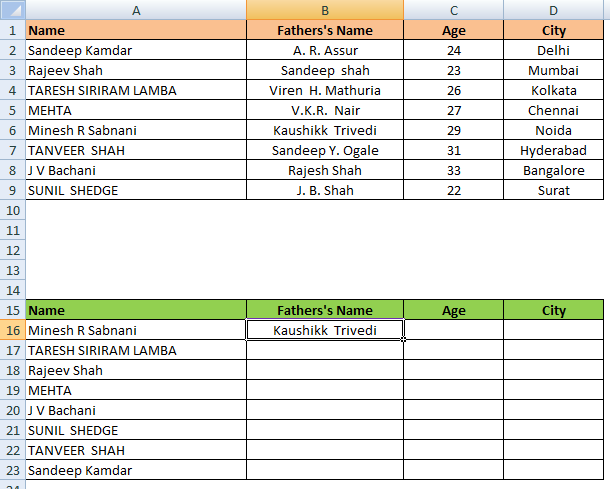This is a Data Sheet from which we have to take data to the other sheet by vlookup formula.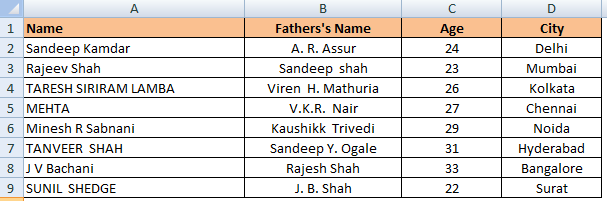This is an excel sheet which have contain only “Name” by which we will fill other all data like Father’s Name, Age & City with the help of vlookup command.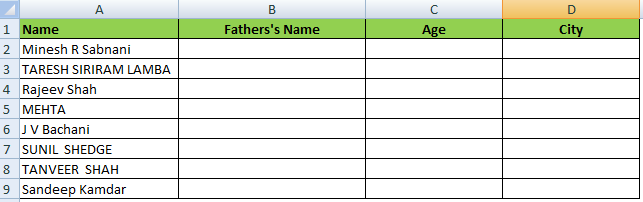Now type the below formula in cell “B2” to get Father’s Name by vlookup formula.

Formula: –    “= VLOOKUP(A16,\$A\$1:\$D\$9,2,0)”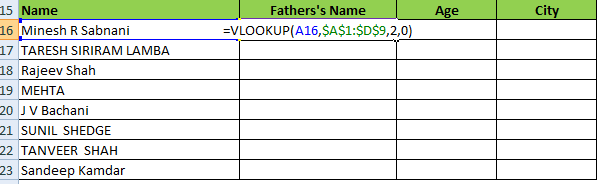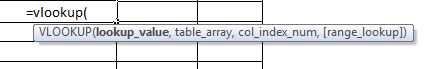Now Drag the Formula in all the cells of column “B” and you will get all father’s name in table will be filled automatically like this-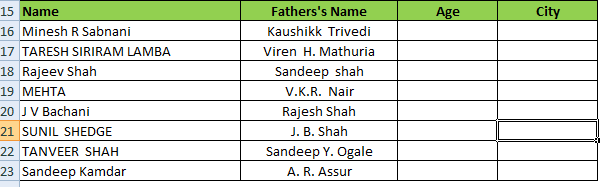Now in the same pattern fill the formula in “Age” Column and “City” Column and all the data will be filled in new sheet automatically.

Check the Formulas filled by me in all the cells:-Now Just Have a look at the result below :-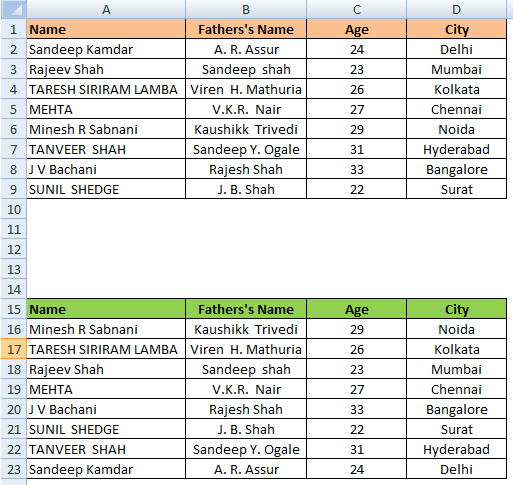All data is filled automatically in  the sheet.

# Method No  2:- Vlookup by Excel Function Box

First of all click on “FX” function button on the top of menu as showing in the image.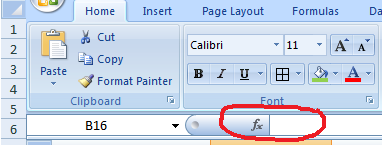Now search vlookup and click on Ok Button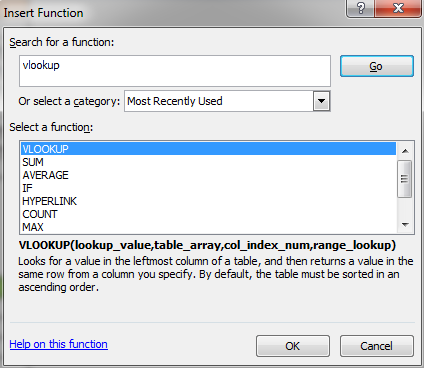Now you can type cell name of you can click on cell to enter the value in function arguments box as under:

Lookup_Value  : A16

Table_array : A1:D9

Col_index_num : 2

Range_lookup : 0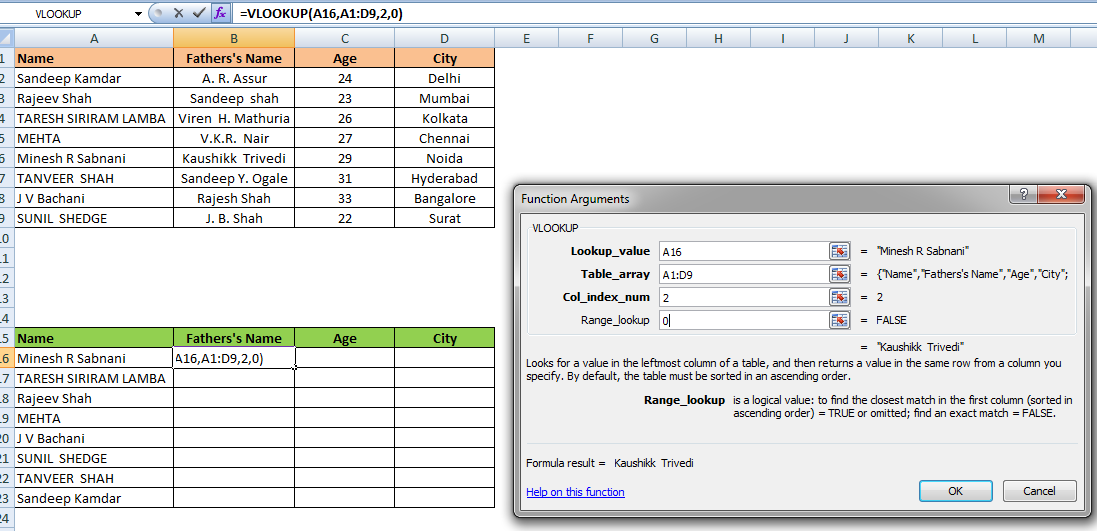And now click on OK Button & you will get the result as under:-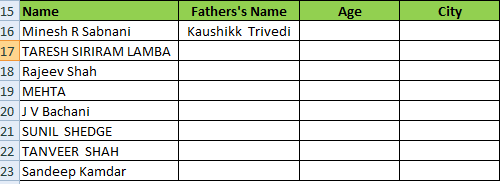Now You can drag the formula or enter one by one with help of Function Argument Box by clicking of function button as show above.

So friends this  was  the Vlookup command that will makes your work so easy and minimize your time of work. If you have any question or suggestion, you can write us in the comment box we will replay your query ASAP.